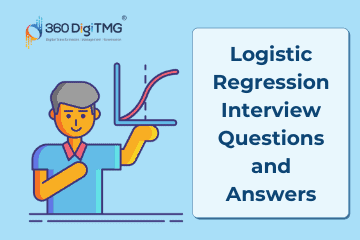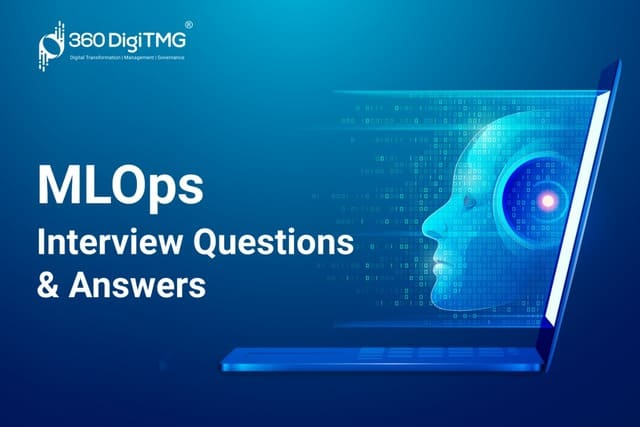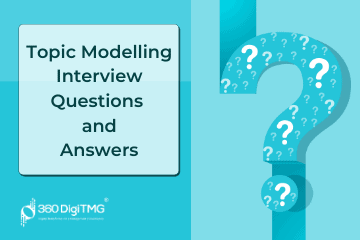Home / Blog / Interview Questions / Logistic Regression Interview Questions & Answers

# Logistic Regression Interview Questions & Answers

• September 02, 2022
• 5387
• 44### Meet the Author : Mr. Bharani Kumar

Bharani Kumar Depuru is a well known IT personality from Hyderabad. He is the Founder and Director of Innodatatics Pvt Ltd and 360DigiTMG. Bharani Kumar is an IIT and ISB alumni with more than 17 years of experience, he held prominent positions in the IT elites like HSBC, ITC Infotech, Infosys, and Deloitte. He is a prevalent IT consultant specializing in Industrial Revolution 4.0 implementation, Data Analytics practice setup, Artificial Intelligence, Big Data Analytics, Industrial IoT, Business Intelligence and Business Management. Bharani Kumar is also the chief trainer at 360DigiTMG with more than Ten years of experience and has been making the IT transition journey easy for his students. 360DigiTMG is at the forefront of delivering quality education, thereby bridging the gap between academia and industry.

• ### Classification analysis is a process of finding a function which divides the dataset into ______ based on different _________?

• a) Dependent based on different Independent
• b) Classes based on different Parameters
• c) Both 1 and 1
• d) None of the above

Answer - b) Classes based on different Parameters

• ### Using ____________ we build the ROC curve (Receiver Operator characteristic) curve.

• a) Confusion matrix
• b) Akaike Information Criterion (AIC) value
• c) Multiple AIC Values
• d) None of the above

• ### What type of chart should we use, if we have estimated a set of data and want to plot the uncertainty of estimation?

• a) Heat map
• b) Contour plot
• c) Error bar plot
• d) 3D Surface

Answer - c) Error bar plot

• ### Which of the following is the true statement for supervised Models? Statement 1: Classification analysis is a process of finding a function that divides the dataset into classes based on different parameters. Statement 2: Regression analysis is a process of finding the correlation between dependent and independent variables in predicting a continuous value.

• a) Statement 1 is true and statement 2 is false
• b) Statement 1 is False and statement 2 is true
• c) Both Statement (1 & 2) is wrong
• d) Both Statement (1 & 2) is true

Answer - d) Both Statement (1 & 2) is true

• ### Which of the following is a good dataset characteristic?

• a) Large enough to yield meaningful result
• b) Is representative of the dataset as a whole
• c) Both 1 and 2
• d) None of the above

Answer - c) Both 1 and 2

• a) True
• b) False

• ### When performing regression or classification, which of the following is the right way to process the data?

• a) Normalize -> PCA -> Training
• b) PCA -> Normalize -> Training
• c) Normalize -> PCA -> Normalize PCA o/p -> Training
• d) None of the above

Answer - a) Normalize -> PCA -> Training

• ### Increase the number of training examples in logistic regression will eventually decrease the Bias and increase the variance?

• a) True
• b) False
• c) None of the above
• d) The given statement is wrong

• ### Application of where classification is used?

• a) Customer churn prediction
• b) Handwriting Recognitions
• c) Breast cancer Detection
• d) All of the above

Answer - d) All of the above

• ### Both Null & Residual deviance is called _________.

• a) Underfitting
• b) Overfitting
• c) Goodness of fit
• d) None of the above

Answer - c) Goodness of fit

• a) True
• b) False

• ### A supervised learning (discrete target) model, also referred to as a classification model, can be evaluated using metrics such as _______?

• a) R Square, Adjusted R square, MSPE and MSAE
• b) Precision, Recall, Accuracy and ROC-AUC
• c) CV error, BLEU Score
• d) Mutual Information

Answer - b) Precision, Recall, Accuracy and ROC-AUC

• ### What is the output of the following? x = [‘ab’, ‘cd’] y= (tuple(x)) print(x,y)

• a) [‘ab’, ‘cb’] (‘ab’, ‘cd’)
• b) ([‘a’, ‘b’], [‘c’, ‘d’])
• c) ([‘a’, ‘b’], [‘c’, ‘d’])
• d) [[‘a’, ‘b’,‘c’, ‘d’]]

Answer - a) [‘ab’, ‘cb’] (‘ab’, ‘cd’)

• ### Which of the following is a true statement for logistic Regression? How to measure the accuracy of logistic regression? Statement 1: Root means the square error is used to measure the accuracy of the classification problems Statement 2: Where the prediction is < 0.5 there the predicted variable = 0. Where the prediction is >= 0.5 there the predicted variable = 1.

• a) Statement 1 is true and statement 2 is false
• b) Statement 1 is False and statement 2 is true
• c) Both Statement (1 & 2) is true
• d) None of them

Answer - b) Statement 1 is False and statement 2 is true

• ### What are the assumptions of logistic regression?

• a) Linearity
• b) No endogeneity, No autocorrelation
• c) Normality and homoscedasticity, No multicollinearity
• d) All the above

Answer - d) All the above

• ### F1 Score is used to measure?

• a) Test’s accuracy
• b) Train accuracy
• c) Validation data
• d) All the above